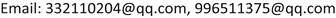Infinite Abel Groups Having Only One Infinite Subgroup<sup> </sup>

Qi Deng, Nuo Li

School of Mathematics, Yunnan Normal University, Kunming YunnanReceived: Dec. 11th, 2020; accepted: Jan. 8th, 2021; published: Jan. 15th, 2021ABSTRACT

In this paper, we study a class of infinite Abel group, which has only one infinite subgroup, that is, the group itself. Here we first discuss the structure and properties of this Abel group, and then give two theorems related to this group, and the corresponding proofs of the theorems.

Keywords:Isomorphism, Cyclic P-Group, Infinite Group, Finite Subgroup1. 引言

2. 预备知识

1) ∀ a ∈ Z ( p ∞ ) , | a | = p i , i ∈ Z 。

2) 任取 Z ( p ∞ ) 的真子群H，存在某个整数k使得 H = 〈 1 / p k ¯ 〉 ，自然的，所有 Z ( p ∞ ) 的真子群都是有限群。

3) 任取 Z ( p ∞ ) 的真子群H，有 Z ( p ∞ ) / H ≅ Z ( p ∞ ) 成立。

3. 证明

1) 先证 ∪ n = 1 ∞ G n = G ： ∀ x ∈ G l ⊂ ∪ n = 1 ∞ G n ， ∀ y ∈ G m ⊂ ∪ n = 1 ∞ G n ，不妨认为 m > l ，则 x ∈ G m ，所以 x − y ∈ G m ⊂ ∪ n = 1 ∞ G n ，所以 ∪ n = 1 ∞ G n 是G的子群，而 ∪ n = 1 ∞ G n 不是有限群，所以由定义 ∪ n = 1 ∞ G n = G 。

2) 对所有的n， G n 都是循环P-群：对包含链 G 1 ⊂ G 2 ⊂ ⋯ ⊂ G n ⊂ ⋯ ，把这些群都分解为循环P-群的内直积，如果某一项 G k ，可以被分解为两个或以上循环P-群的内直积，不妨记为： G k = G a ⋅ G b ，对任意包含 G k 的群 G m ，必然有 G a ⊂ G m ， G b ⊂ G m ， G a ∩ G b = e ，利用有限Abel群分解定理， G m 的分解式中也必然直接或间接的包含 G a 和 G b 这两个循环P-群，记为： G m = G A ⋅ G B ⋅ G m 1 ⋯ G m l ，其中 G a ⊂ G A ， G b ⊂ G B ，通过这种分析也可以得到推论，分解式的长度是单调递增的。模仿分析学中对无限的定义，考虑分解式的长度(或者说符号 ⋅ 的个数)是否会随着m的增加而趋于无穷，分为两种情况讨论：

G k = G k 1 ⋯ G k n 中，利用前面的分析知道有 G ( k + 1 ) i ⊇ G k i 成立，所以 | G k i | 随着m的增加单调递增，又考虑到 lim k → ∞ | G k | = lim k → ∞ | G k 1 | ⋯ ⋅ | G k n | = ∞ ，这式子意味着必然有某项 | G k i | 随着m的增加趋于无穷，不妨认为 lim k → ∞ | G k 1 | = ∞ ，考虑集合 H = ∪ k = m ∞ G k 1 ，由包含关系 G k 1 ⊂ G ( k + 1 ) 1 ⊂ G ( k + 2 ) 1 ⊂ ⋯ 可以推出H是一个无限群，而又有 ( | G m 2 | , | G k 1 | ) = 1 ，所以 G m 2 ⊈ G k 1 ，进一步可以推出 G m 2 ⊈ H ，又因为 G m 2 是G的子群，所以 H ≠ G ，推出矛盾。

3) G = ∪ n = 1 ∞ G n ≅ Z ( p ∞ ) ：因为 G n ⊂ G n + 1 ⋯ 是一列循环P-群，在同构意义下可以用模n加法群来代替表示它们，记做 Z | G n | ⊂ Z | G n + 1 | ⊂ ⋯ ，为了使这个包含关系成立，我们认为 k ¯ ∈ Z | G n | 和 | G n + 1 | | G n | ⋅ k ¯ ∈ Z | G n + 1 | 是同一个元素。

1) f是一个映射。

2) f是满射。因为 ( n ¯ a , n ¯ b ) → f n a + b a ⋅ b ¯ ，利用简单的数论知识知道a和b互素可以推出 a + b 和 a × b 互素，这意味着元素 n ⋅ a + b a ⋅ b ¯ 会随着n的变化而取遍 0 a ⋅ b ¯ 到 a ⋅ b − 1 a ⋅ b ¯ 的所有元。

3) f是单射。 c = 0 ¯ 的充分且必要条件是 a ⋅ b | x ⋅ b + y ⋅ a ，因此 x = 0 ( mod a ) ， y = 0 ( mod b ) ，故 ker f = ( 0 ¯ , 0 ¯ ) 。